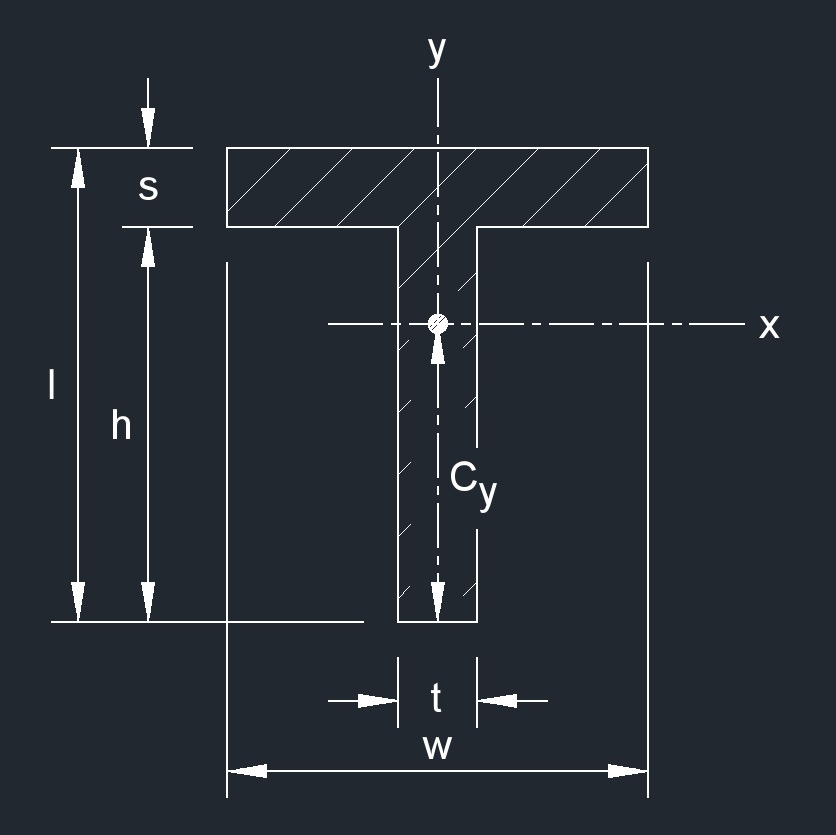# Square T Beam

Written by Jerry Ratzlaff on . Posted in Structural

## Square T Beam - Geometric Properties### area of a Square T Beam formula

$$\large{ A = ws + ht }$$

### Perimeter of a Square T Beam formula

$$\large{ P = 2 \left( w + h + s \right) }$$

### Distance from Centroid of a Square T Beam formula

$$\large{ C_x = 0 }$$

$$\large{ C_y = \frac { l^2t + s^2 \left( w - t \right) } { 2 \left( ws + ht \right) } }$$

### Elastic section Modulus of a Square T Beam formula

$$\large{ S_{x} = \frac { I_{x} } { C_{y} } }$$

$$\large{ S_{y} = \frac { I_{y} } { C_{x} } }$$

### Polar Moment of Inertia of a Square T Beam formula

$$\large{ J_{z} = I_{x} + I_{y} }$$

$$\large{ J_{z1} = I_{x1} + I_{y1} }$$

### Radius of Gyration of a Square T Beam formula

$$\large{ k_{x} = \sqrt { \frac { I_{xc} } { A } } }$$

$$\large{ r_{zc}{^2} = k_{xc}{^2} + k_{yc}{^2} }$$

### Second Moment of Area of a Square T Beam formula

$$\large{ I_{x} = \frac { t \left( w - C_y \right)^3 + w \left[ w - \left( w - C_y \right) \right]^3 - \left( w - t \right) \left[ w - \left( w - C_y \right) -t \right]^3 } {3} }$$

$$\large{ I_{x} = \frac { ht^3 } {24} + \frac { w^3 s } {24} }$$

$$\large{ I_{x1} = I_{x} + A C_{y} }$$

$$\large{ I_{y1} = I_{y1} + A C_{x} }$$

### Torsional Constant of a Square T Beam formula

$$\large{ J = \frac { ws^3 + l - \left( \frac {s}{2} \right) t^3 } {3} }$$

Where:

$$\large{ A }$$ = area

$$\large{ C }$$ = distance from centroid

$$\large{ d }$$ = distance from principle axis

$$\large{ I }$$ = moment of inertia

$$\large{ J }$$ = torsional constant

$$\large{ k }$$ = radius of gyration

$$\large{ P }$$ = perimeter

$$\large{ p }$$ = principal axis

$$\large{ S }$$ = elastic section modulus